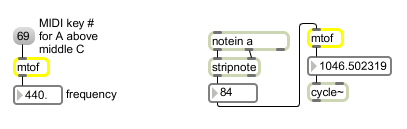# mtof

Convert a MIDI note number to frequency

## Description

mtof performs MIDI-note-number to frequency conversion. Frequency is reported in Hertz (Hz).

None.

## Messages

 int MIDI-note-number (0 through 127) [int] A MIDI note number value from 0 to 127. The corresponding frequency is sent out the outlet. float MIDI-note-number (0 through 127) [float] A MIDI note number value from 0 to 127. The corresponding frequency is sent out the outlet. list MIDI note list [list] A list of MIDI note numbers. Corresponding frequency values for each list item are sent out the outlet.

## Attributes

Name Type g/s Description
base float Sets the "base frequency" used when calculating frequency values (e.g., A = 440.). The default is 440.

## Output

float: The frequency corresponding to the received MIDI pitch value.

## ExamplesUse MIDI note number to provide frequency value for an oscillator